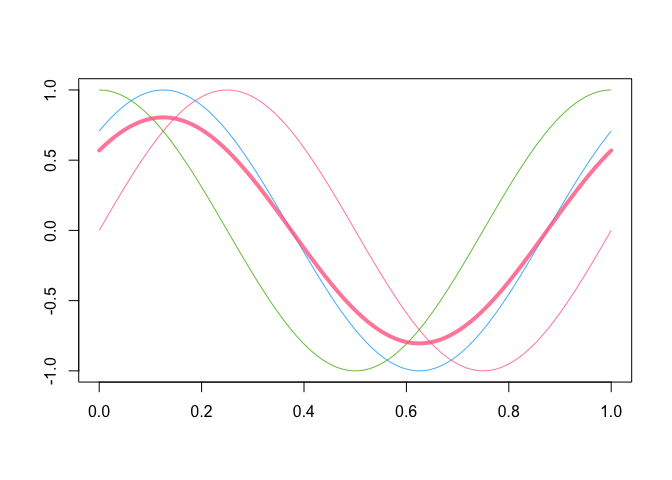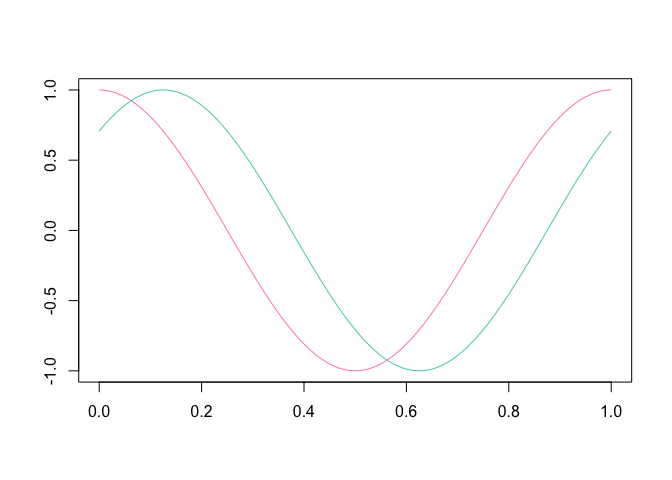# roahdThe roahd (Robust Analysis of High-dimensional Data) package allows to use a set of statistical tools for the exploration and robustification of univariate and multivariate functional datasets through the use of depth-based statistical methods.

In the implementation of functions, special attention was put to their efficiency, so that they can be profitably used also for the analysis of high-dimensional datasets.

For a full-featured description of the package, please take a look at the roahd vignette.

## Installation

Install the released version of roahd from CRAN:

``install.packages("roahd")``

Or install the development version from GitHub with:

``````# install.packages("remotes")
remotes::install_github("astamm/roahd")``````

## `fData` and `mfData` objects

A simple `S3` representation of functional data object, `fData`, allows to encapsulate the important features of univariate functional datasets (like the grid of the dependent variable, the pointwise observations, etc.):

``````library(roahd)

# Grid representing the dependent variable
grid = seq( 0, 1, length.out = 100 )

# Pointwise measurements of the functional dataset
Data = matrix( c( sin( 2 * pi * grid ),
cos ( 2 * pi * grid ),
sin( 2 * pi * grid + pi / 4 ) ), ncol = 100, byrow = TRUE )

# S3 object encapsulating the univariate functional dataset
fD = fData( grid, Data )

# S3 representation of a multivariate functional dataset
mfD = mfData( grid, list( 'comp1' = Data, 'comp2' = Data ) )``````

Also, this allows to exploit simple calls to customized functions which simplifies the exploratory analysis:

``````# Algebra of fData objects
fD + 1 : 100
fD * 4

fD + fD

# Subsetting fData objects (providing other fData objects)
fD[ 1, ]
fD[ 1, 2 : 4]

# Sample mean and (depth-based) median(s)
mean( fD )
mean( fD[ 1, 10 : 20 ] )
median_fData( fD, type = 'MBD' )``````
``````# Plotting functions
plot( fD )
plot( mean( fD ), lwd = 4, add = TRUE )````````plot( fD[ 2:3, ] )``## Robust methods for functional data analysis

A part of the package is specifically devoted to the computation of depths and other statistical indices for functional data:

• Band depths and modified band depths,
• Modified band depths for multivariate functional data,
• Epigraph and hypograph indexes,
• Spearman and Kendall’s correlation indexes for functional data,
• Confidence intervals and tests on Spearman’s correlation coefficients for univariate and multivariate functional data.

These also are the core of the visualization / robustification tools like functional boxplot (`fbplot`) and outliergram (`outliergram`), allowing the visualization and identification of amplitude and shape outliers.

Thanks to the functions for the simulation of synthetic functional datasets, both `fbplot` and `outliergram` procedures can be auto-tuned to the dataset at hand, in order to control the true positive outliers rate.

## Citation

If you use this package for your own research, please cite the corresponding R Journal article:

``````To cite roahd in publications use:

Ieva, F., Paganoni, A. M., Romo, J., & Tarabelloni, N. (2019). roahd
Package: Robust Analysis of High Dimensional Data. The R Journal,
11(2), pp. 291-307.

A BibTeX entry for LaTeX users is

@Article{,
title = {{roahd Package: Robust Analysis of High Dimensional Data}},
author = {Francesca Ieva and Anna Maria Paganoni and Juan Romo and Nicholas Tarabelloni},
journal = {{The R Journal}},
year = {2019},
volume = {11},
number = {2},
pages = {291--307},
url = {https://doi.org/10.32614/RJ-2019-032},
}``````